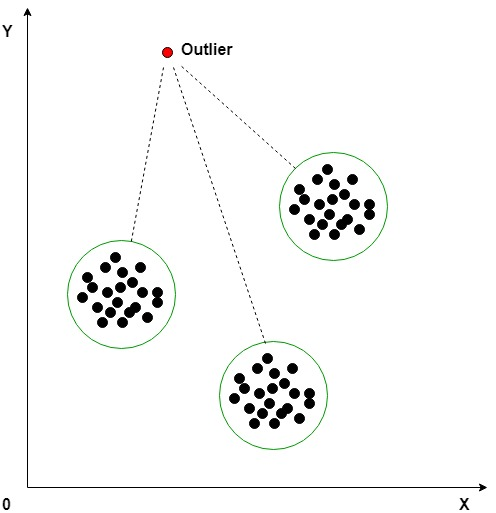# Machine Learning | Outlier

• Difficulty Level : Easy
• Last Updated : 23 Feb, 2020

An outlier is an object that deviates significantly from the rest of the objects. They can be caused by measurement or execution error. The analysis of outlier data is referred to as outlier analysis or outlier mining.

Why outlier analysis?
Most data mining methods discard outliers noise or exceptions, however, in some applications such as fraud detection, the rare events can be more interesting than the more regularly occurring one and hence, the outlier analysis becomes important in such case.Detecting Outlier:
Clustering based outlier detection using distance to the closest cluster:
In the K-Means clustering technique, each cluster has a mean value. Objects belong to the cluster whose mean value is closest to it. In order to identify the Outlier, firstly we need to initialize the threshold value such that any distance of any data point greater than it from its nearest cluster identifies it as an outlier for our purpose. Then we need to find the distance of the test data to each cluster mean. Now, if the distance between the test data and the closest cluster to it is greater than the threshold value then we will classify the test data as an outlier.

Algorithm:

1. Calculate the mean of each cluster
2. Initialize the Threshold value
3. Calculate the distance of the test data from each cluster mean
4. Find the nearest cluster to the test data
5. If (Distance > Threshold) then, Outlier
My Personal Notes arrow_drop_up